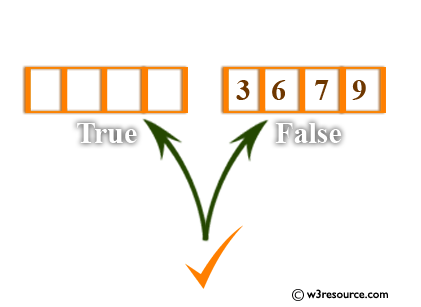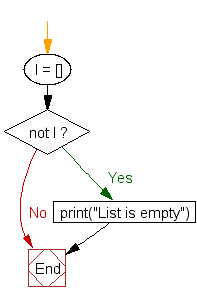﻿ Python: Check a list is empty or not - w3resource# Python: Check a list is empty or not

## Python List: Exercise-8 with Solution

Write a Python program to check a list is empty or not.Sample Solution:-

Python Code:

``````l = []
if not l:
print("List is empty")
```
```

Sample Output:

```List is empty
```

Flowchart:## Visualize Python code execution:

The following tool visualize what the computer is doing step-by-step as it executes the said program:

Python Code Editor:

Have another way to solve this solution? Contribute your code (and comments) through Disqus.

What is the difficulty level of this exercise?

Test your Python skills with w3resource's quiz

﻿

## Python: Tips of the Day

Floor Division:

When we speak of division we normally mean (/) float division operator, this will give a precise result in float format with decimals.

For a rounded integer result there is (//) floor division operator in Python. Floor division will only give integer results that are round numbers.

```print(1000 // 300)
print(1000 / 300)```

Output:

```3
3.3333333333333335```